# RBSE Solutions For Class 12 Physics Chapter 12: Nature of Light | Textbook Important Questions & Answers

The answers are provided for all the questions of Chapter 12 Physics of RBSE Class 12. Students can go through these questions to understand the concepts better and score well in the board examination and entrance examinations for various professional courses. These solutions are provided by the team of experts and offer the best and accurate solutions to the questions. Do check BYJU’S RBSE Class 12 solutions page to get the answers for textbook questions of all subjects.

## Multiple Choice Questions

Q1: To show the phenomenon of interference, we need two sources which emit radiation:

1. Of equal frequency and fixed phase difference
2. Of nearly equal frequency
3. Of equal frequency
4. Of different wavelength

Q2: In Young’s double slit experiment monochromatic light source is used. The shape of the fringes on the screen will be:

1. Straight line
2. Hyperbola
3. Parabola
4. Circle

Q3: In an Interference experiment on using light of wavelength 700 nm, third bright fringe received at a point on the screen. At the same point, to receive fifth bright fringe the wavelength of light is:

1. 210 nm
2. 315 nm
3. 420 nm
4. 490 nm

Q4: In Young’s Double Slit experiment, if the ratio of the widths of slits are 4:9, then the ratio of maximum and minimum frequencies will be:

1. 196 : 25
2. 81 : 16
3. 25 : 1
4. 9 : 4

Q5: In Young’s Double Slit Experiment, the light of two different wavelengths are used. For yellow-orange colour (𝛌 = 600 nm) third-order bright fringe position coincides with the fourth-order bright fringe of the second colour. The wavelength of the second colour will be:

1. 500 nm
2. 450 nm
3. 225 nm
4. 350 nm

Q1: The line perpendicular to a wavefront gives the direction of which quantity?

Answer: It gives the direction of the ray.

Q2: Which physical quantities affect the width of Young’s fringes?

Answer: The physical quantities like wavelength, the distance of slit from the screen and the distance between the slits.

Q3: Write the principle for Huygen’s principle for diffraction of light?

Answer: The diffraction is the result of the superposition of secondary waves starting from the different points of the same wavefront.

Q4: What type of wavefront emerges from

(i) Point source

(ii) Far light source

(i) Spherical wavefront emerges from a point source

(ii) Plane wavefront emerges from a far light source

Q5: Which is the most important condition for the interference of two waves?

Answer: The most important condition for the interference of two waves is that both sources of light should be coherent.

Q6: How does the angular separation between fringes change in a single slit diffraction experiment when the distance between the slit and screen doubled?

Answer: The relation between angular separation and changes in slit separation is given by

θ∝1/D, from the equation we understand that when the value of D is doubled then the angular separation between the fringes decreases by half

Q7: For clear diffraction of waves what should be the range of size of obstacle or hole?

Answer: The size of the obstacle is of the order of wavelength.

Q8: Write the expression of two physical events which proves the wave nature of light?

Answer: Interference and polarisation proves the wave nature of light

Q9: Why does light seem to propagate in a straight line although it is of wave nature?

Answer: That is because the wavelength of light is very small.

Q10: In an experiment for diffraction through a hole, which light waves superimpose?

Answer: The slit becomes a source of secondary wavelets. These secondary wavelets superimpose.

Q11: Write down the mathematical form of Malus law?

Answer: Mathematical expression for Malus law is

I = I0cos2θ

Here I is the light after leaving the second polaroid, I0 is the maximum intensity before entering the second polaroid, θ is the angle between planes of polarizer and analyzer.

Q1: Give Huygen’s principle for light waves?

Huygen’s Principle is also called as Huygen’s – Fresnel principle. According to Huygen’s Principle, each point on the given wavefront is a source of secondary wavelets or secondary disturbances. Further, the secondary wavelets travel in the forward direction at the same speed as the source wave. The new wavefront will be a line tangent of the secondary wavelets.Huygen’s Principle can be explained using the given formula.

s = vt

Where,

s is the distance,v is the speed and t is the time

From the equation, we can understand that the wavefront emits waves with the speed equal to v. The wavelets emitted are semicircular in shape and it occurs after a time t. The new wavefront is the tangent to the wavelets.

Q2: Define interference of waves

Interference of light is the phenomenon of modification in the intensity of light due to the superposition of two or more waves. When light waves from two or more coherent sources superpose, the distribution of energy will not be uniform. At some points, two crests or two troughs superimpose which increases the amplitude to a maximum resulting in maximum intensity. This type of interference is called constructive interference. At certain points, a crest and a trough superpose and the amplitude decreases to the minimum or zero resulting in minimum or zero intensity. This type of interference is called destructive interference.

Q3: What do you mean by coherent sources?

Two sources are said to be coherent if the waves emitted from them have the same frequency and a constant phase difference. Laser light is an example of coherent light.

Q4: What do you understand by the diffraction of light? Compare the diffraction of light waves and sound waves?

Diffraction is the slight bending of light as it passes around the corners of the obstacle or through the slit into the region of the geometric shadow of the obstacle.

The diffraction is seen more in sound waves than in light waves. The reason is that light waves have a very small wavelength compared to that of sound waves. It is necessary for the wavelength of waves to be greater than the size of the opening before diffraction can occur, it is then clear that light waves will only be diffracted by very small openings while sound waves suffer diffraction even on wider openings.

Q5: Define resolving power of a microscope. How is it affected

1. When the wavelength of incident radiation is reduced.
2. When the diameter of the objective lens is reduced. Give the reason for your answer.

Resolving power is defined as the inverse of the distance or angular separation between two objects which can be just resolved when viewed through the optical instrument.

For microscopes, the resolving power is the inverse of the distance between two objects that can be just resolved. This is given by the famous Abbe’s criterion given by Ernst Abbe in 1873 as

Δd = λ/2n sin θ

Resolving power =1/Δd = 2nsin θ/λ

Where n is the refractive index of the medium separating object and aperture. Note that to achieve high-resolution n sin θ must be large. This is known as the Numerical aperture.

1. Since resolving power ∝ 1/λ, when the wavelength of the incident radiation is reduced resolving power increases.
2. When the aperture of the objective lens decreases, the semi-vertical angle θ decreases and hence the resolving power increases.

Q6: Fringes are formed on screen due to interference of light from two thin slits. If the distance between slits rises four times and the distance of the screen from slits is halved then how many times will be the fringe width?

Answer: The fringe width is given by the expression

β = Dλ/2d

If the distance between the slits d increased by 4 times and the distance of the screen from the slit is halved then the value of β is given by

β’ =

β’ =

Therefore β’ =(⅛)β

Q7: Explain the construction of polaroid.

Answer: Polaroids are a large-sized film kept between two glass plates. The film is prepared by taking very small sized crystals of quinine or sulphate and setting them on a sheet of Nitro-cellulose such that the optic axis remains parallel to each other. These crystals absorb one of the two refracted rays and the second plane polarised ray has emerged. Every polaroid has a characteristic direction known as ”Polarisation Direction”.

When a beam of light passes through the polaroid, the vibrations parallel to the polarising direction emerge and all other vibrations are absorbed. The emerging ray is plane-polarized.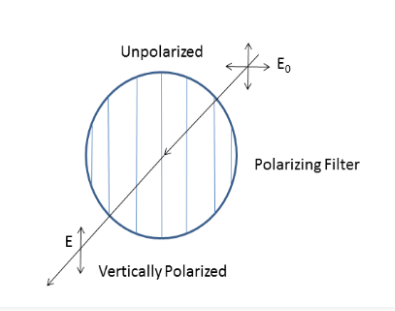Q8: What do you mean by double refraction?

Double refraction is an optical property in which a single ray of unpolarized light entering a medium is split into two rays, each travelling in a different direction. The principle of double refraction is used in a Nicol prism. The Nicol prism is made using Calcite crystal pieces. The calcite crystal is cut into two pieces at a certain angle and joined using a transparent cement called Canada Balsam.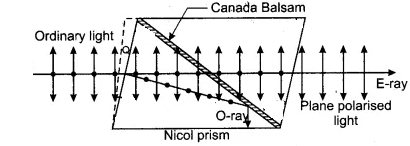Q9: Write the differences between interference and diffraction.

 Interference Diffraction Interference occurs between waves emerging from two different sources, producing different wavefronts. Diffraction occurs between different secondary waves that emerge from the different parts of the same wave. Fringe with is generally constant Fringes are of varying width All the positions in bright fringes are of the same intensity Intensity varies There is a good contrast between the Maxima and Minima There is poor contrast between the Maxima and Minima It has a large number of fringes It has less number of fringes

Q10: Write down the main differences between Fresnal and Fraunhofer diffraction.

 Fresnel Diffraction Fraunhofer Diffraction The source and the screen are situated near the obstacle causing diffraction The source and the screen are far from the obstacle causing diffraction The beam of light is not modified by a lens A convex lens is used to produce a diffraction pattern The wavefronts are spherical or cylindrical Incident wavelengths on the diffracting obstacle are plane

## Essay Type Questions

Q1: On the basis of Huygen’s wave principle, give the interpretation of the reflection of light.

Huygen’s principle also sometimes called the Huygens–Fresnel principle states that each point on a given wavefront is a source of secondary wavelets or secondary disturbances. Further, the disturbances originating from the secondary source spreads in all directions in the same way as originating from the primary source.

Interpretation of the reflection of light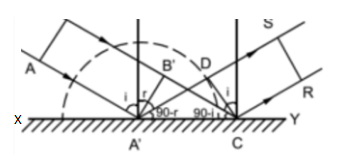If you look at the figure, AA’ is the wavefront incident on a reflecting surface XY having an angle of incidence i. Following Huygen’s principle, every point on AA’ will act as a source of secondary wavelets.

Time taken to move from A’ to D = time taken to move from B’ to C

A’D / v = B’C / v

A’D = B’C

A’C sin(i) = A’C sin(r)

Thus, i = r

Hence, the angle of incidence and angle of reflection are both equal. This is also stated by the first law of reflection. Additionally, as the incident wavefront AB, the normal and reflected wavefront is on the same plane, we can also verify the second law of reflection.

Q2: Write down analytical explanation of interference and write down conditions for constructive and destructive interference.

Interference is what happens when two or more waves meet each other. Depending on the alignment of crests and troughs of the overlapping waves, they might add up, or they can partially or entirely cancel each other.

If the crest of a wave meets the crest of another wave of the same frequency at the same point, then the resultant amplitude is the sum of individual amplitudes – this is known as constructive interference. Similarly, if the crest of a wave meets the trough of another wave, then the resultant amplitude is equal to the difference in the individual amplitudes – this is known as destructive interference. The formula for the sum of two waves can be derived as follows:

The amplitude of a sinusoidal wave travelling to the right along the x-axis is given by,

W1(x,t) = Acos(kx – ωt)

Where A is the peak amplitude, k = 2π/λ is the wavenumber and ω = 2πf is the angular frequency of the wave.

Consider another wave of the same frequency and amplitude but with a different phase travelling to the right.

W2(x,t) = Acos(kx – ωt+Φ)

where φ is the phase difference between the waves in radians

The two waves superimpose and add; the resultant wave is given by the equation,

W1(x,t) + W2(x,t) = A[cos(kx – ωt) + cos(kx – ωt+Φ)]—————————(1)

The sum of two cosines is given by the equation,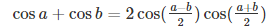Solving equation (1) using the formula, we get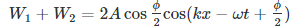Constructive Interference: When the phase difference is an even multiple of π (φ = …..,–4π, –2π, 0, 2π, 4π,……), then cos φ/2 =1, so the sum of the two waves is a wave with twice the amplitude.

W1+W2 = 2Acos(kx – ωt)

Destructive Interference: When the phase difference is an odd multiple of π (φ =…..,–3π, –π, 0, π, 3π, 5π,……), then cos φ/2 = 0, so the sum of the two waves will be zero.

W1+W2 = 0